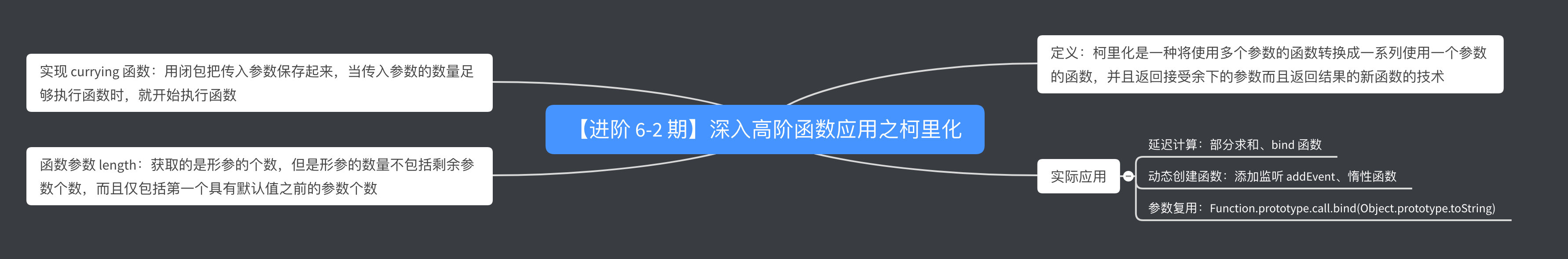# # 深入高阶函数应用之柯里化

## # 引言## # 柯里化

### # 定义

``````// 木易杨
const add = (...args) => args.reduce((a, b) => a + b);

// 假设我们实现了一个 currying 函数，支持一次传入一个参数
// 封装第一个参数，方便重用
``````

### # 实际应用

#### # 1、延迟计算

``````// 木易杨
const add = (...args) => args.reduce((a, b) => a + b);

// 简化写法
function currying(func) {
const args = [];
return function result(...rest) {
if (rest.length === 0) {
return func(...args);
} else {
args.push(...rest);
return result;
}
}
}

sum(1,2)(3); // 未真正求值
sum(4); 		 // 未真正求值
sum(); 			 // 输出 10
``````

``````// 木易杨
let obj = {
name: 'muyiy'
}
const fun = function () {
console.log(this.name);
}.bind(obj);

fun(); // muyiy
``````

``````// 木易杨
// 简化实现，完整版实现中的第 2 步
Function.prototype.bind = function (context) {
var self = this;
// 第 1 个参数是指定的 this，截取保存第 1 个之后的参数
// arr.slice(begin); 即 [begin, end]
var args = Array.prototype.slice.call(arguments, 1);

return function () {
// 此时的 arguments 是指 bind 返回的函数调用时接收的参数
// 即 return function 的参数，和上面那个不同
// 类数组转成数组
var bindArgs = Array.prototype.slice.call(arguments);
// 执行函数
return self.apply( context, args.concat(bindArgs) );
}
}
``````

#### # 2、动态创建函数

``````// 简化写法
function addEvent (type, el, fn, capture = false) {
}
else if(window.attachEvent){
el.attachEvent('on' + type, fn);
}
}
``````

``````const addEvent = (function(){
return function (type, el, fn, capture) {
}
}
else if(window.attachEvent){
return function (type, el, fn) {
el.attachEvent('on' + type, fn);
}
}
})();
``````

``````function addEvent (type, el, fn, capture = false) {
// 重写函数
addEvent = function (type, el, fn, capture) {
}
}
else if(window.attachEvent){
addEvent = function (type, el, fn) {
el.attachEvent('on' + type, fn);
}
}
// 执行函数，有循环爆栈风险
}
``````

#### # 3、参数复用

``````function isArray(obj) {
return Object.prototype.toString.call(obj) === '[object Array]';
}

function isNumber(obj) {
return Object.prototype.toString.call(obj) === '[object Number]';
}

function isString(obj) {
return Object.prototype.toString.call(obj) === '[object String]';
}

// Test
isArray([1, 2, 3]); // true
isNumber(123); // true
isString('123'); // true
``````

``````const toStr = Function.prototype.call.bind(Object.prototype.toString);

// 改造前
[1, 2, 3].toString(); // "1,2,3"
'123'.toString(); // "123"
123.toString(); // SyntaxError: Invalid or unexpected token
Object(123).toString(); // "123"

// 改造后
toStr([1, 2, 3]); 	// "[object Array]"
toStr('123'); 		// "[object String]"
toStr(123); 		// "[object Number]"
toStr(Object(123)); // "[object Number]"
``````

### # 实现 currying 函数

``````// 木易杨
function currying(fn, length) {
length = length || fn.length; 	// 注释 1
return function (...args) {			// 注释 2
return args.length >= length	// 注释 3
? fn.apply(this, args)			// 注释 4
: currying(fn.bind(this, ...args), length - args.length) // 注释 5
}
}

// Test
const fn = currying(function(a, b, c) {
console.log([a, b, c]);
});

fn("a", "b", "c") // ["a", "b", "c"]
fn("a", "b")("c") // ["a", "b", "c"]
fn("a")("b")("c") // ["a", "b", "c"]
fn("a")("b", "c") // ["a", "b", "c"]
``````
• 注释 1：第一次调用获取函数 fn 参数的长度，后续调用获取 fn 剩余参数的长度

• 注释 2：currying 包裹之后返回一个新函数，接收参数为 `...args`

• 注释 3：新函数接收的参数长度是否大于等于 fn 剩余参数需要接收的长度

• 注释 4：满足要求，执行 fn 函数，传入新函数的参数

• 注释 5：不满足要求，递归 currying 函数，新的 fn 为 `bind` 返回的新函数（`bind` 绑定了 `...args` 参数，未执行），新的 length 为 fn 剩余参数的长度

``````// 参考自 segmentfault 的@大笑平
const currying = fn =>
judge = (...args) =>
args.length >= fn.length
? fn(...args)
: (...arg) => judge(...args, ...arg)

// Test
const fn = currying(function(a, b, c) {
console.log([a, b, c]);
});

fn("a", "b", "c") // ["a", "b", "c"]
fn("a", "b")("c") // ["a", "b", "c"]
fn("a")("b")("c") // ["a", "b", "c"]
fn("a")("b", "c") // ["a", "b", "c"]
``````

``````add(1, 2, 3) // 6
``````

``````function add(a) {
return function(b) {
return a + b;
}
}

``````

``````function add(a) {
return function(b) {
return function (c) {
return a + b + c;
}
}
}
``````

``````// 注释同上
function currying(fn, length) {
length = length || fn.length;
return function (...args) {
return args.length >= length
? fn.apply(this, args)
: currying(fn.bind(this, ...args), length - args.length)
}
}
``````

### # 扩展：函数参数 length

``````((a, b, c) => {}).length;
// 3

((a, b, c = 3) => {}).length;
// 2

((a, b = 2, c) => {}).length;
// 1

((a = 1, b, c) => {}).length;
// 0

((...args) => {}).length;
// 0

const fn = (...args) => {
console.log(args.length);
}
fn(1, 2, 3)
// 3
``````

``````const fn = currying((a = 1, b, c) => {
console.log([a, b, c]);
});

fn();
// [1, undefined, undefined]

fn()(2)(3);
// Uncaught TypeError: fn(...) is not a function
``````

### # 小结

• 定义：柯里化是一种将使用多个参数的函数转换成一系列使用一个参数的函数，并且返回接受余下的参数而且返回结果的新函数的技术
• 实际应用
• 延迟计算：部分求和、bind 函数
• 参数复用：`Function.prototype.call.bind(Object.prototype.toString)`
• 实现 currying 函数：用闭包把传入参数保存起来，当传入参数的数量足够执行函数时，就开始执行函数
• 函数参数 length：获取的是形参的个数，但是形参的数量不包括剩余参数个数，而且仅包括第一个具有默认值之前的参数个数

## # 参考资料

JavaScript 专题之函数柯里化

JavaScript 专题之惰性函数